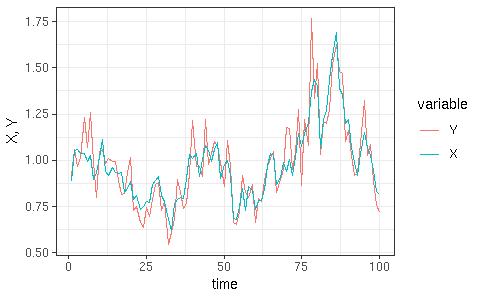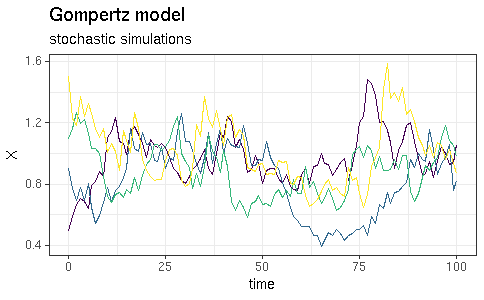# Coding POMP models: R vs C snippets

#### 30 July 2021

This document was produced using pomp version 3.5.0.2 and R version 4.1.0.

To implement models in pomp, we have various options. While it is very easy to code basic model components using R functions, it can be much faster to do it using C snippets. Here, we’ll demonstrate both approaches.

library(pomp)
library(ggplot2)
library(magrittr)

The Gompertz model is a very simple partially observed Markov process (POMP) model that we’ll use to demonstrate.

The latent state variable, $$X$$ represents the density of a population of biological organisms. The state equation is $X_{t+\delta} = K^{1-S}\,X_{t}^S\,\epsilon_{t},$ where $$S=e^{-r\,\delta}$$ is a parameter and the $$\epsilon_t$$ are i.i.d. lognormal random deviates with variance $$\sigma^2$$: $\epsilon_t \sim \mathrm{Lognormal}(0,\sigma).$ The observed variables $$Y_t$$ are lognormally distributed: $Y_t \sim\mathrm{Lognormal}(\log{X_t},\tau).$

## Implementation using R functions

To begin with, we’ll code the latent state process model and both components of the measurement model using R functions.

simulate(times=1:100,t0=0,
params=c(K=1,r=0.1,sigma=0.1,tau=0.1,X.0=1),
rprocess=discrete_time(
step.fun=function (X,r,K,sigma,...,delta.t) {
eps <- exp(rnorm(n=1,mean=0,sd=sigma))
S <- exp(-r*delta.t)
c(X=K^(1-S)*X^S*eps)
},
delta.t=1
),
rmeasure=function (X, tau, ...) {
c(Y=rlnorm(n=1,meanlog=log(X),sdlog=tau))
},
dmeasure=function (tau, X, Y, ..., log) {
dlnorm(x=Y,meanlog=log(X),sdlog=tau,log=log)
}
) -> gompertz

We plot the results.

gompertz %>%
as.data.frame() %>%
melt(id="time") %>%
ggplot(aes(x=time,y=value,color=variable))+
geom_line()+
labs(y="X, Y")+
theme_bw()## Implementation using C snippets

Now we’ll code up the same example using C snippets.

simulate(times=0:100,t0=0,
params=c(K=1,r=0.1,sigma=0.1,tau=0.1,X.0=1),
dmeasure=Csnippet("
lik = dlnorm(Y,log(X),tau,give_log);"
),
rmeasure=Csnippet("
Y = rlnorm(log(X),tau);"
),
rprocess=discrete_time(
step.fun=Csnippet("
double S = exp(-r*dt);
double logeps = (sigma > 0.0) ? rnorm(0,sigma) : 0.0;
X = pow(K,(1-S))*pow(X,S)*exp(logeps);"
),
delta.t=1
),
paramnames=c("r","K","sigma","tau"),
obsnames="Y",
statenames="X"
) -> Gompertz

## Testing the implementations

Having coded the model, it’s a good idea to run some simple tests to check the implementation. We can run simulations and a particle filter operation to check that the rprocess, rmeasure, and dmeasure components function without error.

First, some simulations, from several initial conditions:

p <- parmat(coef(Gompertz),4)
p["X.0",] <- c(0.5,0.9,1.1,1.5)
simulate(Gompertz,params=p,format="data.frame") %>%
ggplot(aes(x=time,y=X,group=.id,color=.id))+
geom_line()+
guides(color=FALSE)+
theme_bw()+
labs(title="Gompertz model",subtitle="stochastic simulations")We run 10 replicate particle filter operations at the true parameter values as follows. This allows us to assess the Monte Carlo error in the likelihood estimate.

pf <- replicate(n=10,pfilter(Gompertz,Np=500))

logmeanexp(sapply(pf,logLik),se=TRUE)
##                    se
## 62.8137837  0.1679958

## Comparing the implementations

Using each implementation, we’ll now run a number of simulations and compare the amount of time required.

system.time(simulate(gompertz,nsim=10000,format="arrays"))
##    user  system elapsed
##   6.916   0.005   6.921
system.time(simulate(Gompertz,nsim=10000,format="arrays"))
##    user  system elapsed
##   0.243   0.004   0.246
system.time(pfilter(gompertz,Np=10000))
##    user  system elapsed
##   6.182   0.002   6.185
system.time(pfilter(Gompertz,Np=10000))
##    user  system elapsed
##   0.289   0.000   0.288